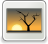# Association of hypertension with risk factors using logistic regression

Mustafa, Mamat and Wan Muhamad Amir, Wan Ahmad and Mohamad Arif, Awang Nawi (2014) Association of hypertension with risk factors using logistic regression. Applied Mathematical Sciences, 8 (49). pp. 2563-2572. ISSN 13147552 [P]Image FH02-FIK-14-00735.jpg Restricted to Registered users only Download (217kB)

## Abstract

Hypertension is one of the important public health challenges worldwide because of its high frequency and concomitant risks of cardiovascular and kidney disease. A hypertension model is built to test the interaction and significance between the factors. In this present paper, we present the results that gained from multiple logistic regression method and used to model the relationship between the ordinal outcome variable. The significant variables is chosen based on the p-value associated to the significant level of model that lies on α = 0.05. Logit determination and the correlation between the variables are also discussed for further analysis. there are three factors that most significant of the six factors tested were identified as having influence significantly the performance of human blood pressure (hypertension). These factors are age (p-value <0.000), body mass index (p-value <0.001) and systolic (p-value <0.001). The use of mathematical software PASW version 18 is applied in this research as an alternatives calculation procedures derived from the methodology.

Item Type: Article Hypertension, Logistic Regression, multiple logistic regression, risk factors and blood pressure R Medicine > R Medicine (General) Faculty of Informatics & Computing Syahmi Manaf 13 Sep 2022 04:32 13 Sep 2022 04:32 http://eprints.unisza.edu.my/id/eprint/5082View Item